• 利用matplotlib做数据可视化
2022-04-11 22:25:23

## matplotlib的作用

使用matplotlib库可以绘制折线图、散点图、条形图、直方图将数据进行可视化，更直观地呈现数据，使数据更加客观更具说服力。

再清楚一下绘图思路：先绘制草图再逐步设置完善图。

## 导入库

在命令执行窗口输入 pip install matplotlib 进行库的导入。

## 简单绘制

首先进行简单绘制，我们需要从matplotlib库中导入pyplot模块，并进行简化命名

以下以绘制折线图为例，散点图和条形图的使用近似，主要区别在于散点图使用plt.scatter()，条形图使用plt.bar()，直方图使用plt.hist() （直方图一般是对没有进行统计过的数据才能使用）

from matplotlib import pyplot as plt
plt.plot()     #图形绘制
plt.show()     #图形展示

## 细节完善

### 设置图片大小

fig = plt.figure(figsize=(20,8),dpi=80)
#通过实例化一个figure并且传递参数，能够在后台自动使用该figure实例
#在图像模糊是可以传入dpi参数，让图片更加清晰
plt.savefig(“./sig_size.png”)#保存在当前路径下并命名为sig_size.png


### 设置x轴和y轴的刻度

x轴和y轴刻度的设置有多种方法：

plt.xticks(x)#直接传入x的数值

plt.xticks(range(2,25))#传入2-24

_xtick_labels = [i for i in range(2,25)]
plt.xticks(_xtick_labels)

#如果刻度太过密集则可以改变步长，间隔取值
plt.xticks(x[::2])

#同样也可以设置y轴的刻度
plt.yticks(range (min(y),max(y)+1))

### 绘制网格

对绘制的图我们还可以添加网格线，对alpha参数进行赋值可以设置网格线透明度。

plt.grid(alpha=0.4)  #alpha设置网格线透明度

### 解决中文显示问题

为了图像便于人们阅读，我们绘图时还需要加入中文，但matplotlib在默认的情况下中文是无法显示的。此时我们就需要找到源码（matplotlib.rc选中在ctrl+B）并进行修改，也有多种方法。

我们可以通过fc-list :lang=zh查看linux/mac下面支持的字体（注意冒号前有空格）

#方法1：查看源码直接复制font并进行修改，此方法在每次绘图前就要修改好

'''
font = {'family' : 'monospace',
'weight' : 'bold',
'size'   : 'larger'}
'''
#以上是原始代码
#修改过后的：
font = {'family': 'MicroSoft YaHei',
'weight': 'bold',
'size': '12'}  #注意字体大小需要使用数字来设置
matplotlib.rc("font",**font)
#或者直接传入到rc中
matplotlib.rc("font",family='MicroSoft YaHei',weight='bold',size='12')

#方法2
from matplotlib import font_manager
My_font = font_manager.FontProperties(fname=”字体文件路径”)
##win字体路径：fname=“\Windows\Fonts\msyh.ttc”(具体名称属性里找)

#方法3
plt.rcParams[“font.sans-serif”]=[“SiHei”]

### 添加描述信息（若中文显示使用方法2需要传入中文字体）

plt.xlabel(“xxx”)   #添加x轴的描述信息
plt.ylabel(“xxx”)   #添加y轴的描述信息
plt.title(“xxx”)    #添加标题

### 添加图例

当我们需要在同一个图中绘制两条及以上的折线时，我们需要对折线进行修饰以区分这两条线

plt.plot(x,y,label=”xx”,color=’r’,linestyle=’--’,linewidth=5)
#传入x，y使其对应起来，命名为xx,设置为宽度为5的红色虚线
plt.legend()  #添加图例
#还可以改变图例的位置
plt.legend(loc=0)   #图例置于最佳位置
plt.legend(loc=upper left)  #将图例置于左上角

如果要改变图例位置则需要查看源码并修改，点击legend，可以看到legend字样高亮，ctrl+B一次后再次找到legend再ctrl+B找到图片所示的legend，然后找到location就可以找到想要的位置的字符串表达或者代码，传入plt.legend()就可以了。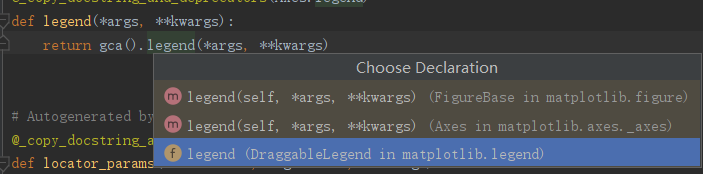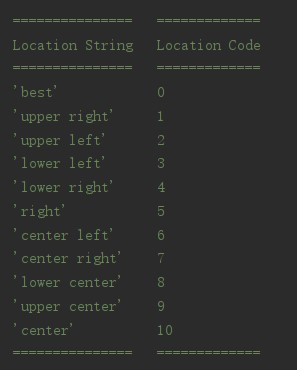### 对折线的个性化设置

 颜色字符 r g b w c m y k 颜色 红色 绿色 蓝色 白色 青色 洋红 黄色 黑色

还可以百度颜色的16进制

 字符 - -- -， ： ‘’ 样式 实线 虚线，破折线 点划线 点虚线，虚线 留空或空格，无线条

以下就是以绘制折线图为例进行一个完整的代码实现

from matplotlib import pyplot as plt
import matplotlib
font = {'family': 'MicroSoft YaHei',
'weight': 'bold',
'size': '12'}
matplotlib.rc("font",**font)

y_1 = [1,1,1,1,2,0,1,2,3,2,4,1,4,2,4,3,3,1,1,1]
y_2 = [1,0,3,1,2,2,1,0,2,1,2,1,1,3,2,1,1,3,1,1]
x = range(11,31)
plt.figure(figsize=(13,6),dpi=80)
plt.plot(x,y_1,label="自己",color='r',linewidth=3,linestyle='--')
plt.plot(x,y_2,label="同桌",color='g',linewidth=3,linestyle='-')
_xtick_labels = ["{}岁".format(i) for i in x]
plt.xticks(x,_xtick_labels)
plt.xlabel("年龄/岁")
plt.ylabel("一年看的课外书的数量/本")
plt.title("不同年龄段看的课外书的数量")
plt.grid(alpha=0.4)
plt.legend()
plt.savefig("./shu.png")
plt.show()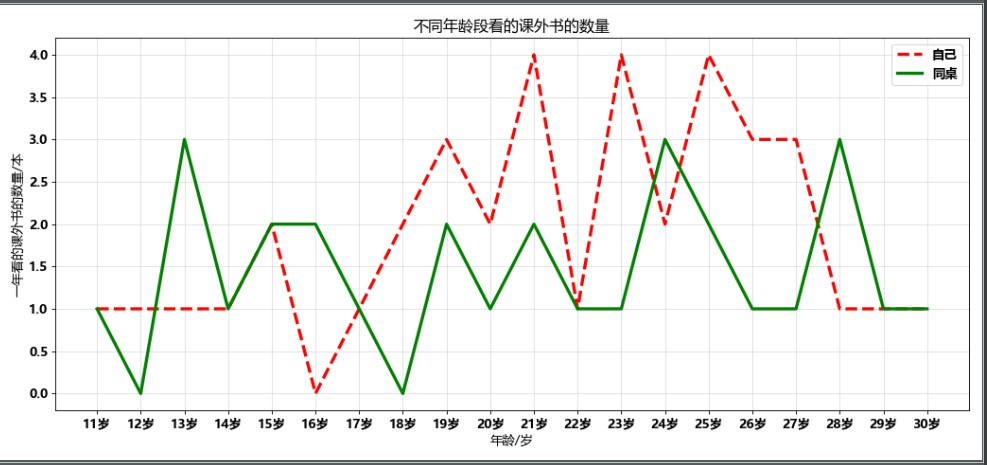python
更多相关内容
• 关于pylab和pyplot，人们过不少的讨论。这两个模块有哪些不同呢？pylab模块跟matplotlib一起安装，而pyplot则是matplotlib的内部模块。两者的导入方法有所不同，可选择其中一种进行导入。 from pylab import * #或...

matplotlib是python中的一个画图库，继承了matlib（从名字上也看得出来）的优点和语法，所以对于熟悉matlib的用户来说是十分友好的。

## pylab和pyplot

关于pylab和pyplot，人们做过不少的讨论。这两个模块有哪些不同呢？pylab模块跟matplotlib一起安装，而pyplot则是matplotlib的内部模块。两者的导入方法有所不同，可选择其中一种进行导入。

from pylab import *
#或
import matplotlib.pyplot as plt
import numpy as np


pylab在同一命名空间整合了pyplot和Numpy的功能，因此无需再单独导入Numpy。更进一步来说，导入pylab后，pyplot和Numpy的函数就可以直接调用，而不用再指定其所属模块（命名空间），从而使得matplotlib开发环境更像是Matlab。

plot(x,y)
array([1,2,3,4])
#而不用指定模块名称
plt.plot()
np.array([1,2,3,4])


大多情况下，我们更乐意使用pyplot模块。

## 线状图

这里我使用jupyter来进行演示

ipython qtconsole --matplotlib inline


用matplotlib生成这个图表很简单，一行代码就能搞定

plt.plot([1,2,3,4])
plt.show()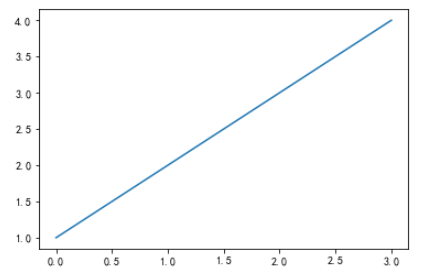如图所示，生成了一个Line2D对象。该对象为一条直线，它表示图表中各数据点的线性延伸趋势。我们可以看出，列表中的数据直接被作为y轴的值展示了出来，x周是从0开始的，所以我们要看一个数据的折线图，只用输入一个list即可。

但我们可以看出这个图可能还很简陋，比如有如下几个问题：

• y轴显示为啥是0.5为步长间隔，我想以1为步长间隔
• 我想控制x轴展示的值，而不是从0开始
• 图太小了，能否控制大小
• x轴和y轴字太小了，能否控制大小
• 给x轴和y轴命个名吧
• 没有网格看不清
• 没有图例
• 给线状图标上点
• 我想保存图片到本地
• 怎么画子图呢

接下来我们就一个一个解决。

### 1.设置x轴和y轴步长间隔

控制x轴，y轴显示的值，有两个参数

• xticks(ticks, [labels], **kwargs)

• yticks(ticks, [labels], **kwargs)

• ticks：控制显示的位置，也就是显示那几个值，这几个值必须在y值数据的范围内，这里也就是[1，4]这个范围。
• [labels]：控制显示在对应位置的值，可以是数也可以是字符。
y = [1,2,3,4]
step = 1
plt.yticks([i for i in y if i%step == 0])
plt.plot(y)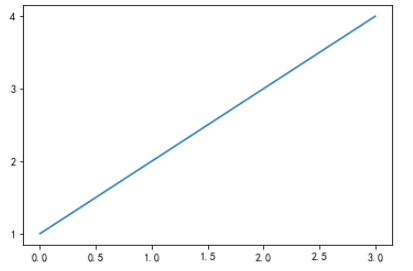### 2.x轴展示的值

这里展示了坐标轴显示字符的情况。

注意第五行，我改成了[1,2,3,3.5,4]，所以显示出来就多了个3.5。

y = [1,2,3,4]
scale_ls = range(4)
index_ls = ["富强","民主","文明","和谐"]
plt.xticks(scale_ls,index_ls)
plt.yticks([1,2,3,3.5,4])
plt.plot(y)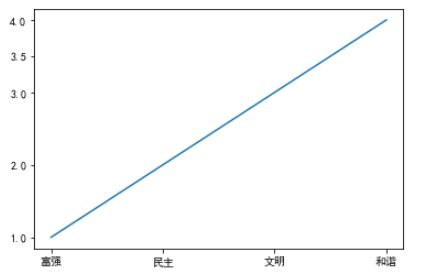### 3.控制图表大小

控制图表的大小要用到的几个方法

• rcParams：这个参数是用来设置一些配置参数的，比如这里我就用到了大小和dpi
• figure.figsize：控制大小，参数为一个二元组(x,y)，即长、宽
• figure.dpi：控制dpi
plt.rcParams['figure.figsize'] = (10,5)
plt.rcParams['figure.dpi'] = 200
y = [1,2,3,4]
scale_ls = range(4)
index_ls = ["富强","民主","文明","和谐"]
plt.xticks(scale_ls,index_ls)
plt.yticks([1,2,3,3.5,4])
plt.plot(y)


这里可以看出更大更清晰了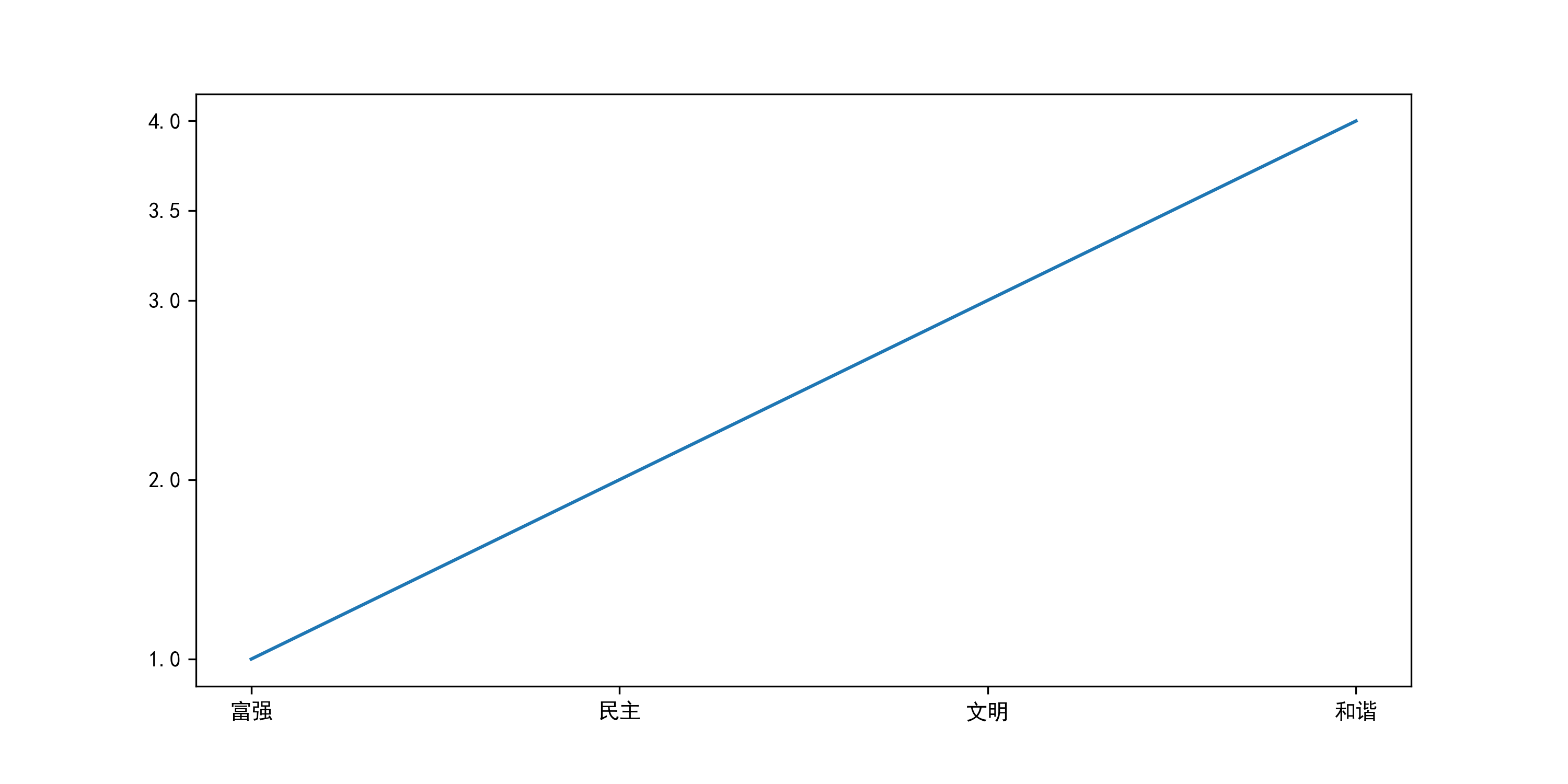### 4.调节x轴和y轴字体大小

这里控制字体大小用到的也是xticksyticks，只不过使用到了fontsize参数。

plt.rcParams['figure.figsize'] = (10,5)
plt.rcParams['figure.dpi'] = 200
y = [1,2,3,4]
scale_ls = range(4)
index_ls = ["富强","民主","文明","和谐"]
plt.xticks(scale_ls,index_ls,fontsize=20)
plt.yticks([1,2,3,3.5,4],fontsize=20)
plt.plot(y)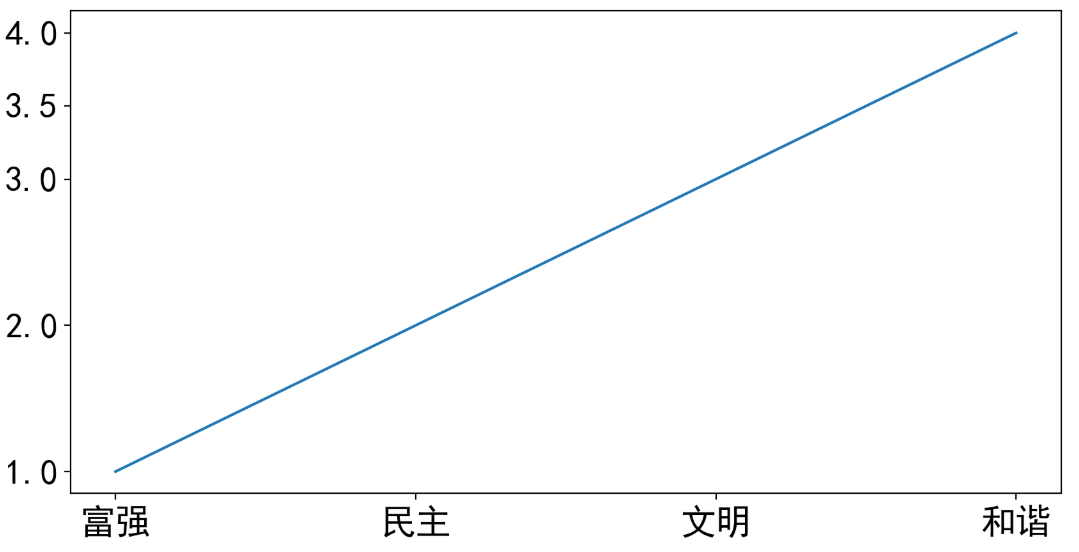### 5.给x轴和y轴加上名字

使用

• xlabel(str,fontsize=int )
• ylabel(str,fontsize=int )
plt.rcParams['figure.figsize'] = (10,5)
plt.rcParams['figure.dpi'] = 200
y = [1,2,3,4]
scale_ls = range(4)
index_ls = ["富强","民主","文明","和谐"]
plt.xticks(scale_ls,index_ls,fontsize=20)
plt.yticks([1,2,3,3.5,4],fontsize=20)
plt.xlabel("核心价值观", fontsize=20)
plt.ylabel("顺序", fontsize=20)
plt.plot(y)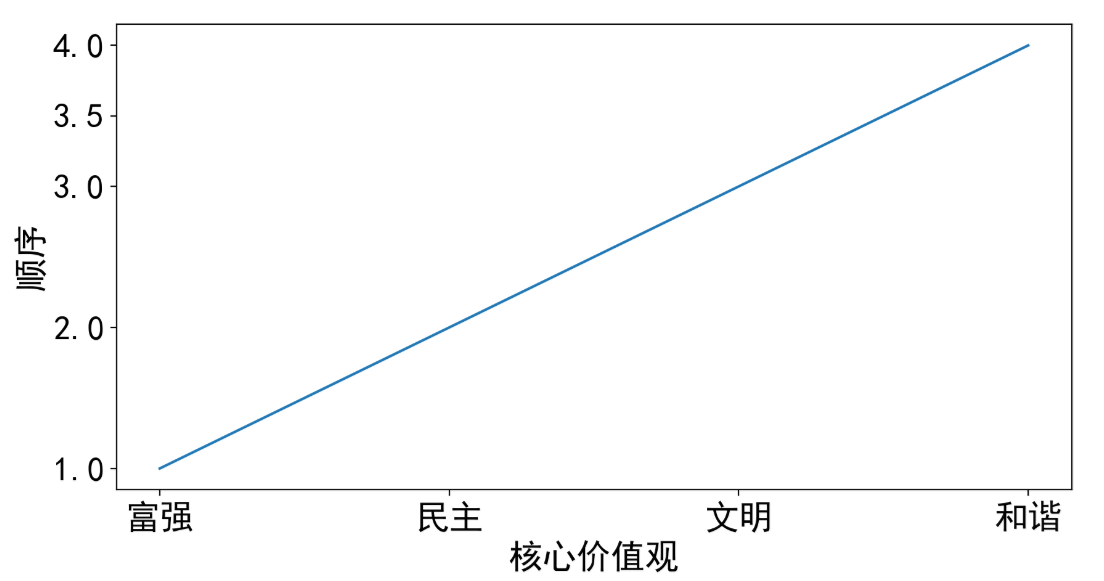### 6.加上网格

• plt.grid(True)，加上横纵两种网格。
• plt.grid(True,axis="x"),加上x轴网格。
plt.rcParams['figure.figsize'] = (10,5)
plt.rcParams['figure.dpi'] = 200
y = [1,2,3,4]
scale_ls = range(4)
index_ls = ["富强","民主","文明","和谐"]
plt.xticks(scale_ls,index_ls,fontsize=20)
plt.yticks([1,2,3,3.5,4],fontsize=20)
plt.xlabel("核心价值观", fontsize=20)
plt.ylabel("顺序", fontsize=20)
plt.grid(True,axis="both")
plt.plot(y)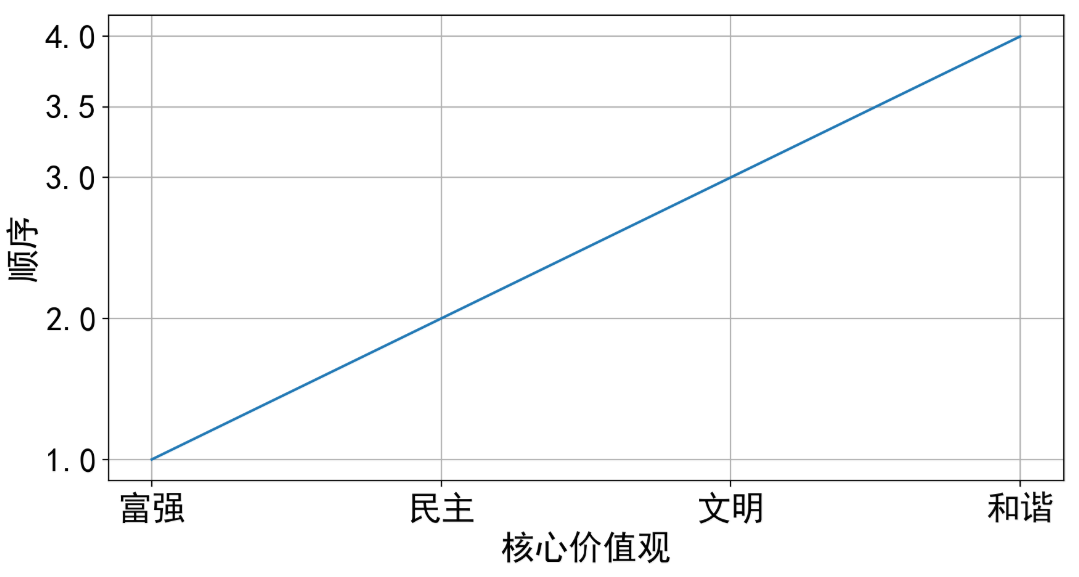### 7.加上图例

使用legend方法，里面有这么几个参数

• handles：代表使用的是那几个曲线的对象
• labels：代表对应的图例文字
• loc：放置的位置
• prop：额外参数，例如size，控制图例大小
t = np.arange(0, 2.5, 0.01)
y1 = map(math.sin, math.pi*t)
y2 = map(math.cos, math.pi*t)
l1, = plt.plot(list(y1))
l2, = plt.plot(list(y2))
plt.legend(handles = [l1, l2], labels = ['Sin', 'Cos'], loc = 'best', prop={'size': 20})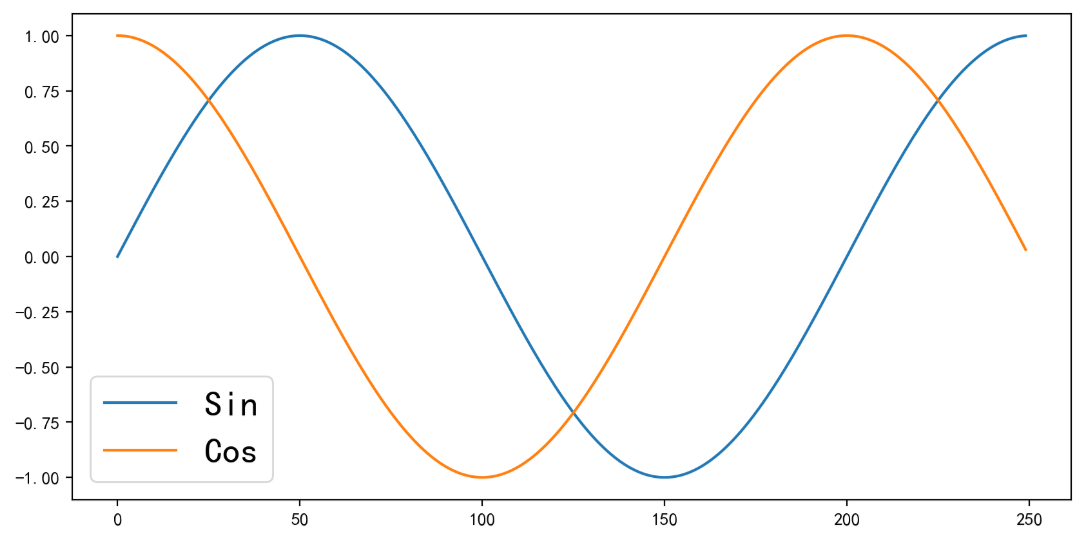### 8.给线状图标点

只用在plot加入参数marker 即可

t = np.arange(0, 2.5, 0.1)
y1 = map(math.sin, math.pi*t)
y2 = map(math.cos, math.pi*t)
l1, = plt.plot(list(y1), marker = "o")
l2, = plt.plot(list(y2), marker = "*")
plt.legend(handles = [l1, l2], labels = ['Sin', 'Cos'], loc = 'best', prop={'size': 20})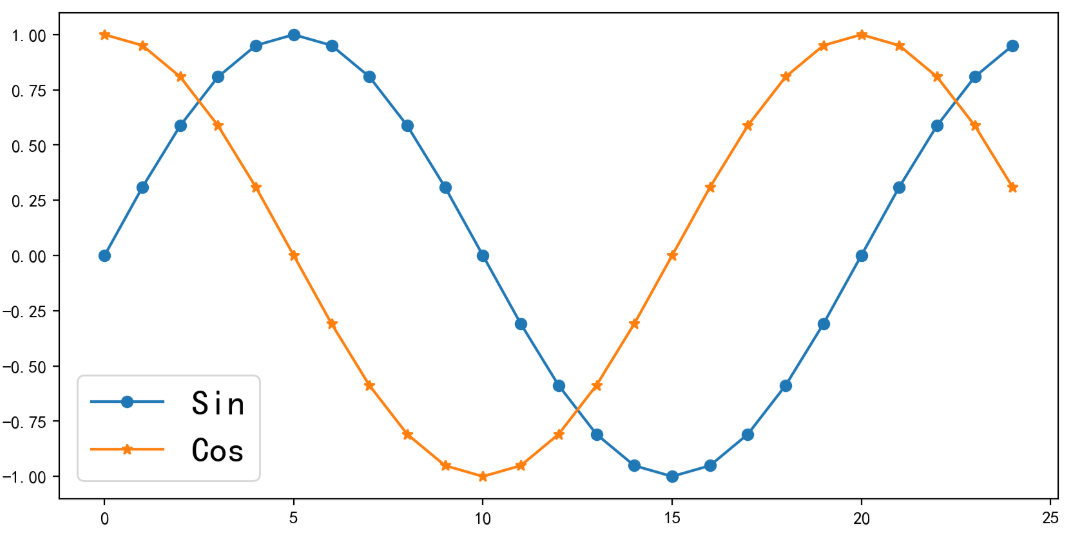### 9.保存图片到本地

只用在最后使用savefig 方法

plt.savefig('test.png',dpi=400)


### 10.画子图

这里使用到了subplot方法

他有三个参数，分别为

• 几行
• 几列
• 第几个

举个栗子

1. subplot(2,2,1) 2行2列（即子图排列为田字格形状）第一个
2. subplot(2,1,2) 2行1列（即子图排列为纵向两个图形状）第二个
t = np.arange(0, 2.5, 0.1)
y1 = map(math.sin, math.pi*t)
y2 = map(math.cos, math.pi*t)

plt.subplot(2, 1, 1)
plt.title("Sin", fontsize=20)
l1, = plt.plot(list(y1), marker = "o")

plt.subplot(2, 1, 2)
plt.title("Cos", fontsize=20)
l2, = plt.plot(list(y2), marker = "*")


这里我还使用到了title方法，给对应图表加上了标题。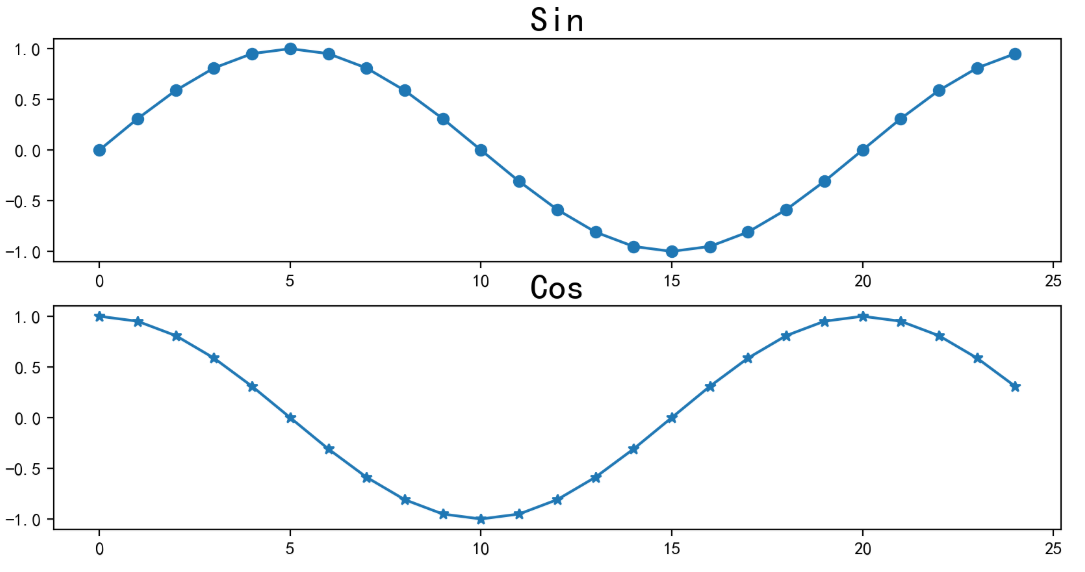展开全文• 接着 使用Matplotlib进行数据可视化(一) ，继续使用 Matplotlib 绘制图像，公众号回复 data2 就可以获得本文章的代码与使用数据。 箱线图(BoxPlot) 箱线图(BoxPlot)也称箱须图(Box-whisker Plot)，它利用数据中的五...
• 利用Matplotlib实现数据可视化（一） 本文首发于微信公众号《Python希望社》，点击这里即可查看原文，欢迎关注，定时持续更新python学习干货。   无论你是编程小白，还是代码高手，大家都应该深有体会，可视化是...

# 利用Matplotlib实现数据可视化（一）

本文首发于微信公众号《Python希望社》，点击这里即可查看原文，欢迎关注，定时持续更新python学习干货。

无论你是编程小白，还是代码高手，大家都应该深有体会，可视化是我们分析数据，做论文报告中不可或缺的一部分。的确，在今天这个时代，大数据与我们的生活息息相关，如何将复杂的数据简洁、明朗的展示在读者面前就显得尤为重要，我们的文章往往最吸引读者的地方也就在于精美的作图。为此，我们推出了python作图系列推文《利用Matplotlib实现数据可视化》。

今天就带大家先来了解一下Matplotlib这个库以及怎么利用Matplotlib作一些简单的图。

## 关于Matplotlib

Matplotlib是一个 Python 的 2D绘图库，它以各种硬拷贝格式和跨平台的交互式环境生成出版质量级别的图形。通过 Matplotlib，开发者可以仅需要几行代码，便可以生成绘图，直方图，功率谱，条形图，错误图，散点图等。

## 使用Matplotlib做一些简单的图

我们日常学习中需要用到的一些基础的图形都可以运用Matplotlib绘图实现，话不多说，接下来就让我们来看几个案例。上图是我最近做的一个数据分析比赛的图，它描述了某天某城市的疫情传播风险区域。利用坐标（原点位置），半径等参数，即可绘制。下面给大家贴上代码。

from pylab import *#调用pylab模块
mpl.rcParams['font.sans-serif'] = ['Microsoft YaHei']
# 输入中文需要用到以上这两行代码
import matplotlib.pyplot as plt#调用Matplotlib.pyplot模块
fig = plt.figure()
#这些是作为单个整数编码的子绘图网格参数。例如，“111”表示“1×1网格，第一子图”，“234”表示“2×3网格，第四子图”。
a1 = Circle(xy=(-4.87, -5.57), radius=1, alpha=0.5, color='red')
a2 = Circle(xy=(-2.53, -0.48), radius=1, alpha=0.5, color='red')
a3 = Circle(xy=(-4.50, -1.16), radius=1, alpha=0.5, color='red')
a4 = Circle(xy=(-5.01, -1.69), radius=1, alpha=0.5, color='red')
#作一个圆，第一个参数为圆心坐标，第二个为半径，第三个为透明度（0-1），第四个表示颜色
plt.axis('scaled')
plt.axis('equal')#x轴与y轴有相同长度
plt.title('疫情传播风险区域')#标题
plt.text(-9.8, -7.5, "单位:千米")#注释，可以放在任何位置
plt.show()


除了画圆，我们还可以如法炮制，绘制一些其他的图形：### Tips

细心的小伙伴可能已经发现了我们在上述案例中用到了两个模块：pylabmatplotlib.pyplot，这里简单的介绍一下这两个模块。

Pyplot：“方便快速绘图matplotlib通过pyplot模块提供了一套和MATLAB类似的绘图API，将众多绘图对象所构成的复杂结构隐藏在这套API内部。”

pylab：“matplotlib还提供了一个名为pylab的模块，其中包括了许多NumPy和pyplot模块中常用的函数，方便用户快速进行计算和绘图，十分适合在IPython交互式环境中使用。”

这里顺带提一下Numpy这个库，Numpy（Numeric Python）是一个模仿matlab的对python数值运算进行的扩展，提供了许多高级的数值编程工具，如：矩阵数据类型、矢量处理，以及精密的运算库。而很多情况下matplot需要配合numpy包一起使用。

作用：pylab = pyplot+大部分numpy。也就是说pylab只是提供了一个方便的导入常用包的接口。

接下来是一些比较实用的作图案例：

（一）柱状图代码如下：

from pylab import *
mpl.rcParams['font.sans-serif'] = ['Microsoft YaHei']
x1 = np.arange(2004, 2017)
y1 = [700, 800, 950, 1100, 1070, 1000, 1050, 900, 950, 900, 1000, 960, 980]
y1 = np.array(y1)
plt.figure(1)
plt.bar(x1, y1, 0.6, color="coral")
plt.title("商品销量与时间的关系")
plt.xlabel(u"年份")
plt.ylabel(u"销量")
plt.text(2001.5, 1100, "单位:个")
plt.show()


（二）折线图代码如下：

from pylab import *
plt.rcParams['font.sans-serif'] = ['Microsoft YaHei']
x1 = np.arange(2004, 2017)
x2 = np.arange(2004, 2017)
y1 = [700, 800, 950, 1100, 1070, 1000, 1050, 900, 950, 900, 1000, 960, 980]
y2 = [500, 600, 650, 600, 670, 700, 750, 800, 650, 500, 700, 760, 680]
y1 = np.array(y1)
y2 = np.array(y2)
plt.figure(1)
plt.plot(x1, y1, label='商品1销量', c='r')
plt.scatter(x1, y1, c='r')
plt.plot(x2, y2, label='商品2销量', c='g')
plt.scatter(x2, y2, c='g')
plt.title("商品销量与时间的关系")
plt.xlabel(u"年份")
plt.ylabel(u"销量")
plt.legend()
plt.xticks(range(2004, 2018, 2))
plt.text(2002, 1150, "单位:个")
plt.show()


（三）饼图代码如下：

from pylab import *
mpl.rcParams['font.sans-serif'] = ['Microsoft YaHei']
def plot_pie(x, y):
colors = 'yellowgreen', 'gold', 'lightskyblue', 'lightcoral', 'red', 'orange', 'blue', 'yellow', 'green',
explode = 0, 0, 0, 0, 0, 0, 0, 0, 0,
plt.pie(y, explode=explode, labels=x, colors=colors, autopct='%1.1f%%', shadow=False, startangle=50)
plt.axis('equal')
plt.show()
if __name__ == '__main__':
result = [5863., 882., 2000., 4470., 1200., 3251., 2480., 2295., 4712]
x = '可口可乐', '毛巾', '牙刷', '食用油', '篮球', '火腿肠', '方便面', '酸奶', '其余'
plot_pie(x, result)


上面的几个例子都是用我们自己得到的数据进行输入作图，下面为大家展示一下利用函数表达式作图。

（四）简单的函数图像代码如下：

python
import matplotlib.pyplot as plt
import numpy as np
x = np.linspace(-1,1,50)
y1 = x ** 2
y2 = x
plt.figure()
plt.plot(x,y1)
plt.figure()
plt.plot(x,y2)
plt.show()
plot as plt
import numpy as np
x = np.linspace(-1,1,50)
y1 = x ** 2
y2 = x
plt.figure()
plt.plot(x,y1)
plt.figure()
plt.plot(x,y2)
plt.show()


以上就是利用Matplotlib绘制的一些最简单也是最常见的图，怎么样，你是否已经开始感受到它的魅力了呢？那么就请期待我们在此系列推文的后续为大家推出更多更精美的绘图吧！

展开全文python
• Matplotlib是使用Python进行数据可视化的基本方法之一，操作简便快捷并且具有良好的操作系统兼容性和图形显示底层接口兼容性，对于数据分析新手来说，是非常好用的可视化工具。尽管近几年新的可视化工具在源源不断地...

## 前言

本篇为《使用Python进行数据分析》中介绍Matplotlib库的基础使用方法的第一篇，主要内容为使用Matplotlib库的一些常用技巧，绘画简易的线形图、散点图、密度图和等高线图等用图，以及进行可视化异常处理等。
Matplotlib是使用Python进行数据可视化的基本方法之一，操作简便快捷并且具有良好的操作系统兼容性和图形显示底层接口兼容性，对于数据分析新手来说，是非常好用的可视化工具。尽管近几年新的可视化工具在源源不断地进入大家的视野中，例如Seaborn、ggplot、HoloViews、AItair以及Pandas中对Matplotlib的API封装用法。但Matplotlib仍是可视化工具中的基础，并且掌握Matplotlib更能让你灵活地控制最终的图形结果，学习Matplotlib仍是学习可视化技术中不可或缺的一环。

## 1.Matplotlib常用技巧

### 1.1 导入Matplotlib

最常用的导入方式

import matplotlib as mpl
import matplotlib.pyplot as plt


### 1.2 设置绘图样式

在Matplotlib中可以使用plt.style来选择一种绘图风格，例如选择经典风格(classic)，可以使用plt.style.available来查看可以使用的库中自带的风格，还可以自定义自己想要的绘图风格，有关绘图风格的详细使用方法会在后续介绍。

两种风格的对比

plt.style.use('classic')
x=np.linspace(0,10,100)
plt.plot(x,np.sin(x))

plt.style.use('bmh')
x=np.linspace(0,10,100)
plt.plot(x,np.sin(x))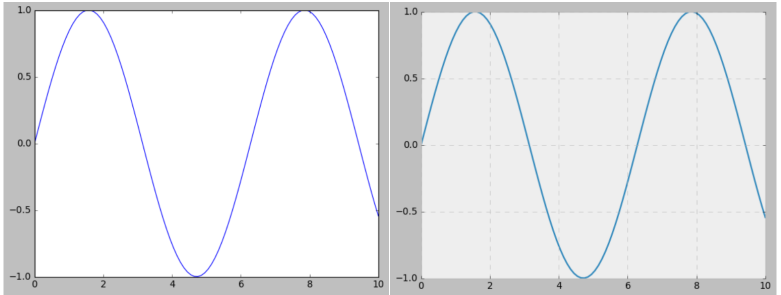### 1.3 show() or not?

在脚本文件中画图时，需要使用plt.show()来显示图形，需注意的是plt.show()通常需要放到脚本的最后，用来显示脚本的绘图。
但如果你使用IPython交互绘图，则不需使用show()命令。使用魔法命令%matplotlib启动Matplotlib模式后即可开始画图。

%matplotlib
Using matplotlib backend: TkAgg

import matplotlib.pyplot as plt


此后的任何plt命令都会自动打开一个图形窗口，增加新的命令就会使图形更新。对于不会即时更新的命令可以使用plt.draw()强制更新。

### 1.4 将图形保存为文件

我们可以使用plt.savefig()命令将绘制的图形保存为文件,且可以指定图片的分辨率、边缘的颜色等参数，具体的使用方法可以参考这篇博文：Python中Matplotlib Savefig()不同参数的使用.

## 2.两种绘图接口

能够分辨Matplotlib的两种绘图接口是非常重要的。初学者经常会搞混或弄不清楚这是怎么回事,接下来介绍这两种绘图接口：一个是便捷的MATLAB风格接口,另一个是功能更强大的面向对象接口。

#MATLAB接口
x=np.linspace(1,10,100)
plt.figure() #创建图形

#创建两个子图中的第一个,设置坐标轴
plt.subplot(2,1,1) #行,列,子图编号
plt.plot(x,np.sin(x))

#创建两个子图中的第二个,设置坐标轴
plt.subplot(2,1,2)
plt.plot(x,np.cos(x))
plt.rcParams['figure.figsize']=(5.0,3.0)

#面向对象接口
#先创建图形网格
#ax是一个包含两个Axes对象的数组
fig,ax=plt.subplots(2)

#在每个对象上调用plot()方法
ax.plot(x,np.sin(x))
ax.plot(x,np.cos(x))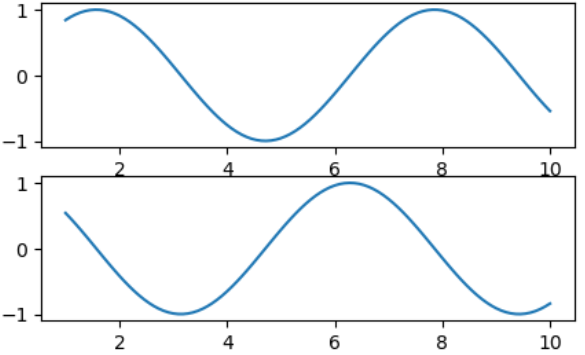MATLAB接口对于初学者来说使用起来会更清楚，更方便而且更快捷。而面向对象的接口则可以适应更复杂的场景，更好地控制你自己的图形。随着后续更深层次内容的介绍，大家会更加了解两种接口的使用以及它们之间的区别和联系。

## 3.绘制简易线形图

现在,让我们来创建一个简单的线形图，即实现线性方程 y = f ( x ) y=f(x) 的可视化。
首先，创建一个图形fig和一个坐标轴ax。

plt.style.use('bmh') #根据自己的喜好设置绘图风格
fig=plt.figure()
ax=plt.axes()


结果如下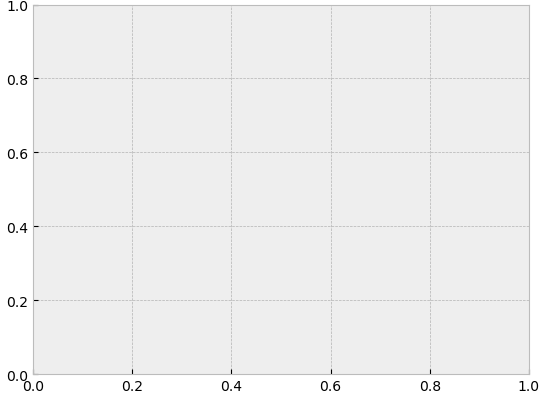在Matplotlib中，figure相当于创建了一张空白的画布，可以容纳各种坐标轴、图形、文字和标签；而axes则是一个带有刻度和标签的矩形，最终会包含所有可视化的图形元素。在创建好坐标轴后，就可以用ax.plot来画图了。

fig=plt.figure()
ax=plt.axes()

x=np.linspace(0,10,1000)
ax.plot(x,np.sin(x))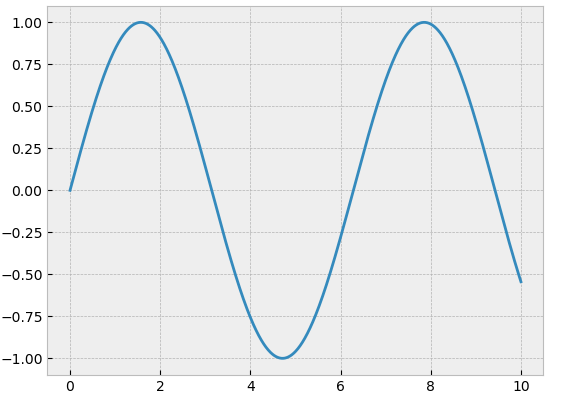另一种绘图方式：

plt.plot(x,np.sin(x)) #直接使用plot命令，不使用面向对象的接口
plt.plot(x,np.cos(x))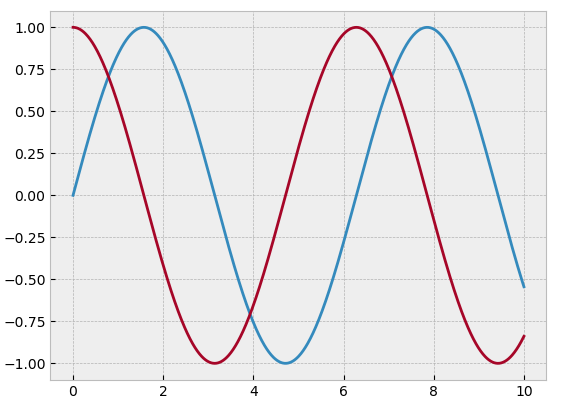### 3.1 调整线条的颜色和风格

首先介绍plot函数中可以进行调整的参数plt.plot(x,y,color,linestyle,marker,alpha,linewidth,markersize)

x,y表示x轴和y轴所对应的数据
color指定线条的颜色
linestyle指定线条类型
marker表示绘制的点的类型
alpha表示点的透明度，用0-1之间的小数表示
linewidth表示线条的宽度，取0-10之间的数值
markersize点的大小

接下来介绍几种常用参数的常用取值
color参数常用的八种取值（颜色）的缩写

颜色缩写代表的颜色颜色缩写代表的颜色
b蓝色g绿色
y黄色m品红
k黑色w白色
r红色c青色

linestyle常用取值

linestyle取值线条风格linestyle取值线条风格
-实线-.点线
- -长虚线:短虚线

线条标记解释

marker取值点的类型marker取值点的类型
‘o’圆圈‘.’
‘D’菱形‘s’正方形
‘h’六边形1‘H’六边形2
‘d’小菱形‘-’水平线
‘v’一角朝下的三角形‘8’八边形
‘<’一角朝左的三角形‘p’五边形
‘>’一角朝右的三角形‘，’像素
‘^’一角朝上的三角形‘+’加号
‘\’竖线‘*’星号
‘x’叉号(X)‘None’

现在举实例说明其具体使用方法

plt.plot(x,np.sin(x),color='blue') #标准颜色名称
plt.plot(x,np.sin(x),color='g') #缩写颜色代码(rgbcmyk)
plt.plot(x,np.sin(x),color='0.75') #范围在0~1之间的灰度值
plt.plot(x,np.sin(x),color='#FFDD44') #十六进制(RRGGBB,00~FF)
plt.plot(x,np.sin(x),color=(1.0,0.2,0.3)) #RGB元组，范围在0~1
plt.plot(x,np.sin(x),color='chartreuse') #HTML颜色名称

plt.plot(x,np.sin(x),linestyle='-') #实线
plt.plot(x,np.sin(x),linestyle='--') #长虚线
plt.plot(x,np.sin(x),linestyle='-.') #点线
plt.plot(x,np.sin(x),linestyle=':') #短虚线


也可以将linestyle和color参数组合起来，用更简洁的表示方式绘图

plt.plot(x,x+1,'-g')
plt.plot(x,x+2,'--c')
plt.plot(x,x+3,'-.k')
plt.plot(x,x+4,':r')


结果如下图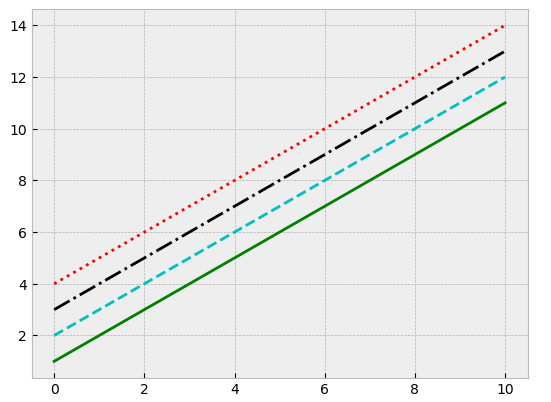### 3.2 调整坐标轴

虽然Matplotlib会为你自动生成坐标轴，但自定义的坐标轴会更符合我们的需求。其最基本的方法是使用plt.xlim()plt.ylim()来调整坐标轴的上下限。

plt.plot(x,np.sin(x))
plt.xlim(-1,11)
plt.ylim(-1.5,1.5)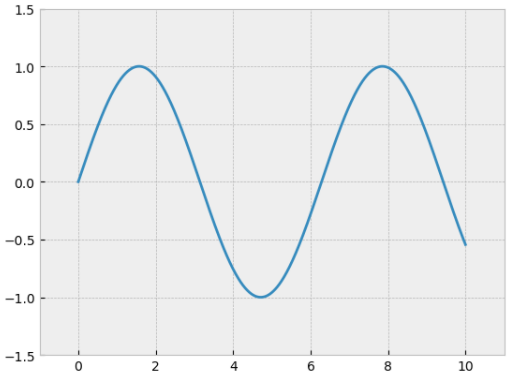如果想要使坐标轴逆序显示，那么也可以逆序设置坐标轴刻度值

plt.plot(x,np.sin(x))
plt.xlim(10,0)
plt.ylim(1.5,-1.5)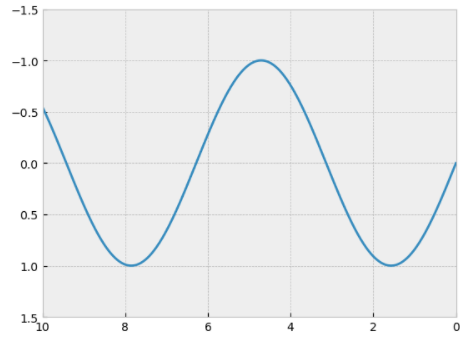再介绍plt.axis()方法，这是一种对坐标轴进行设置的常用方法，例如：

设置坐标轴的上下限plt.axis([-x,x,-y,y])

令图形内容自动紧缩，不留空隙plt.axis('tight)

让x轴与y轴的单位长度相等plt.axis('equal')

开、关坐标轴plt.axis('on'\'off')

等等功能，更详细的使用方法可以查询官网或浏览其他博文，如matplotlib系列-plt.axis

plt.plot(x,np.sin(x))
plt.axis([-1,11,-1.5,1.5])
plt.axis('equal')
plt.axis('off')


结果如下图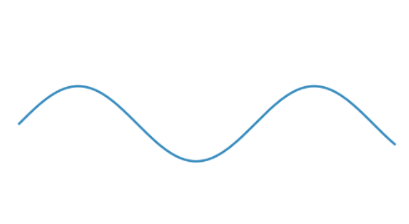### 3.3 设置图形标签

本节简要介绍设置图形标签的方法：图形标题、坐标轴标题、简易图例。
其中图形标题和坐标轴标题是最简单的标签，快速设置方法如下：

plt.plot(x,np.sin(x))
plt.title('A Sine Curve') #设置图形标题
plt.xlabel('x') #设置x轴标题
plt.ylabel('sin(x)') #设置y轴标题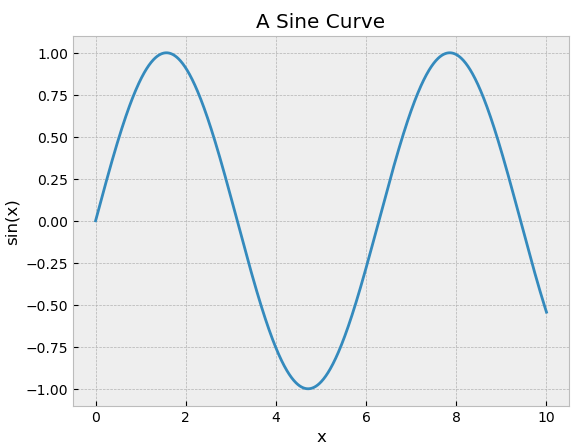我们可以通过调整参数来调整它们的位置，大小和风格。例如size(改变字体大小)、weight(调整字体粗细)和rotation(调整角度)，在此不作过多介绍。

例如

plt.plot(x,np.sin(x))
plt.title('A Sine Curve')
plt.xlabel('x',size=50)
plt.ylabel('sin(x)',rotation=0)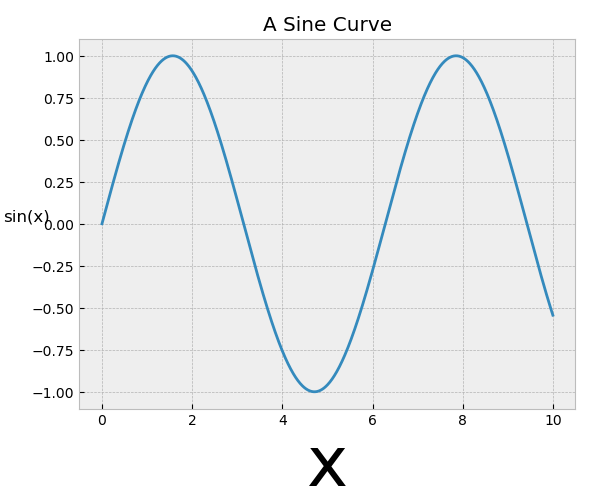在单个坐标轴上同时显示多条线时，创建图例显示每条线是很有效的方法。Matplotlib内置了一个可以用来简单快速创建图例的方法，那就是plt.legend()

plt.plot(x,np.sin(x),'-b',label='sin(x)')
plt.plot(x,np.cos(x),':r',label='cos(x)')
plt.axis('equal')
plt.legend()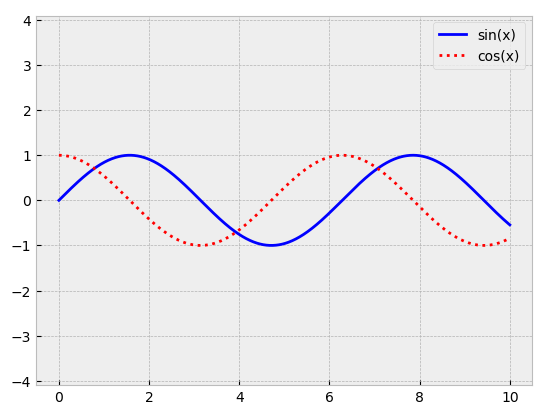在此不再对plt.legend()函数做过多的介绍，在之后的内容中还会介绍更多高级的图例设置方法。

## 4.绘制简易散点图

另一种常用的图形是散点图(scatter plot)，它与线形图类似，只不过不再由线段连接，而是由独立的点、圆圈或是其他形状构成。

### 4.1 使用plt.plot画散点图

与绘制线形图一样，绘制散点图时也可以通过调整参数来自定义绘制的效果，比如颜色和点的形状，用代码示例具体的效果
plt.plot(x,y,marker,color)

rng=np.random.RandomState(0)
for marker in['o','.',',','x','+','v','>','<','^','s','d']:
plt.plot(rng.rand(5),rng.rand(5),marker,label="marker='{0}'".format(marker))
plt.legend(numpoints=1)
plt.xlim(0,1.8)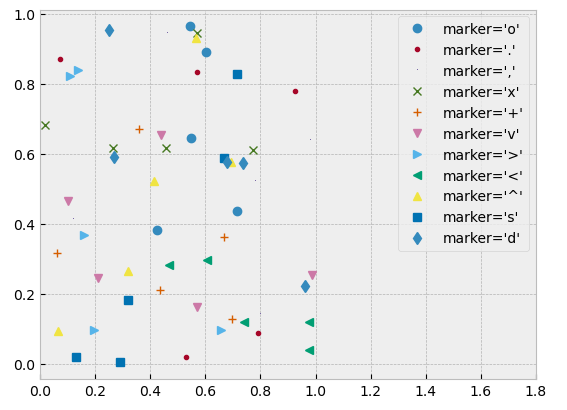我们还可以通过此方法画出一条连接散点的线，并调整它们的参数

plt.plot(x,y,'-*',color='blue',markersize=15,linewidth=3,markerfacecolor='pink',markeredgecolor='blue',markeredgewidth=2)
plt.ylim(-1.2,1.2)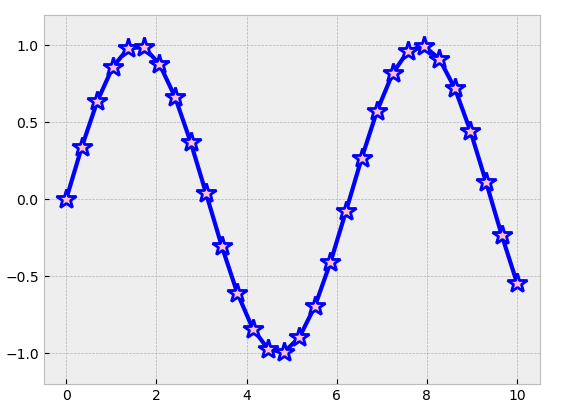### 4.2 使用plt.scatter画散点图

plt.scatter的功能非常强大，它与plt.plot的差别在于它具有更高的灵活性，可以单独控制每个散点与数据匹配，也可以让每个散点具有不同的属性（大小、颜色等）。
plt.scatter(x,y,s,c,marker,alpha)

参数名称说明
x,y表示坐标轴上的数据
s指定点的大小，接收单个值或一维的array数组
c制定点的颜色，接收单个值或一维的array数组
marker表示绘制点的类型
alpha表示点的透明度，范围0-1
plt.style.use('default') #使用原始风格
rng=np.random.RandomState(0)
x=rng.randn(100)
y=rng.randn(100)
colors=rng.rand(100)
sizes=1000*rng.rand(100)
plt.scatter(x,y,c=colors,s=sizes,alpha=0.3,cmap='viridis')
plt.colorbar() #显示颜色条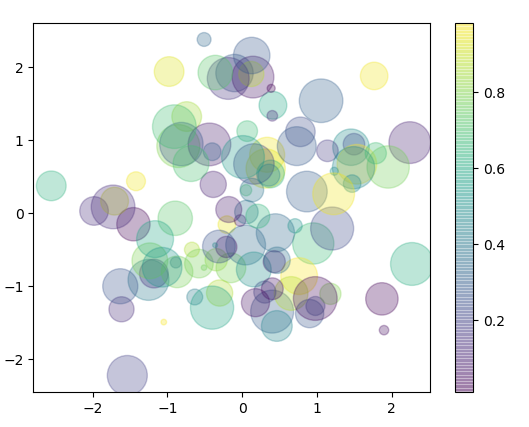散点的颜色和大小可以显示多维数据的信息。让我们举一实例，使用Scikit-Learn程序库中的鸢尾花（iris）数据集来演示。它里面有三种鸢尾花，每个样本是一种花，其花瓣（petal）与花萼（sepal）的长度和宽度都经过了仔细测量。

from sklearn.datasets import load_iris
features=iris.data.T

plt.scatter(features,features,alpha=0.2,s=100*features,c=iris.target,cmap='viridis')
plt.xlabel(iris.feature_names)
plt.ylabel(iris.feature_names)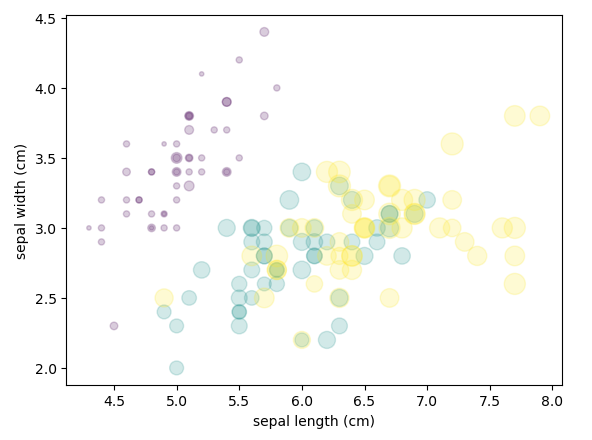由此我们可以看出，散点图可以同时看到不同维度的数据：每个点的坐标值(x,y)分别表示花萼的长度和宽度，而点的大小表示花瓣的宽度，三种颜色对应三种不同类型的鸢尾花。这类多颜色与多特征的散点图在探索与演示数据时非常有用。

### 4.3 plot与scatter的效率对比

由于plt.scatter会对每个散点进行单独的大小和颜色的渲染，因此渲染器会消耗更多的资源。而在plt.plot中，散点基本都彼此复制，因此整个数据集中所有点的颜色、大小都只需要配置一次。因此，在处理大型数据集时它们之间的效率和性能差异就很大了，在面对大型数据集时，plt.plot是更好的方法。当然，例如通过抽样对大型数据集进行处理缩小规模等，就是另外的事情了。

## 5.饼图与条形图

### 5.1 条形图

绘制条形图或说柱状图，我们使用plt.bar()函数，其常用参数表如下
plt.bar(left,height,width=0.8,color)

参数名称说明
left表示x轴的数据
height表示x轴数据的数量
width表示条形图的宽度，范围0-1，默认为0.8
color表示条形图的颜色
x=np.linspace(0,10,10)
plt.bar(x,np.sin(x))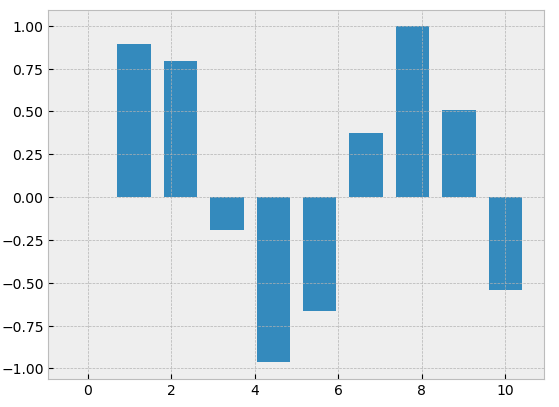x=np.linspace(0,10,30)
plt.bar(x,np.sin(x),color=['red','blue','green'])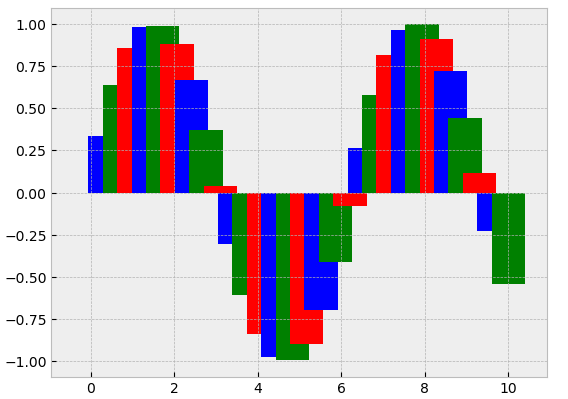若想绘制横向的柱状图，则需使用plt.barh()函数，用法与bar函数相同。

x=np.linspace(0,10,10)
plt.barh(x,np.sin(x))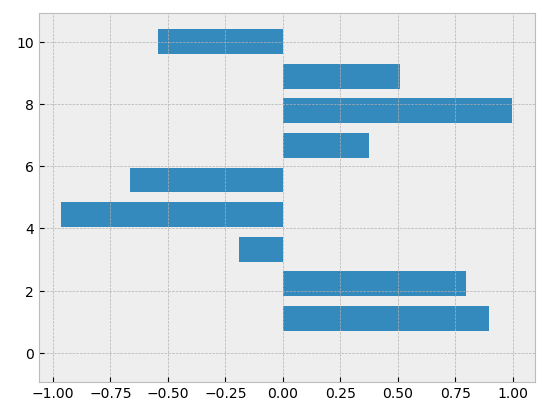我们可以通过使用bottom参数来实现柱状图的堆叠

x=np.linspace(0,10,10)
plt.bar(x,np.sin(x))
plt.bar(x,1.2*np.sin(x),bottom=np.sin(x))
plt.bar(x,1.6*np.sin(x),bottom=np.sin(x)+1.2*np.sin(x))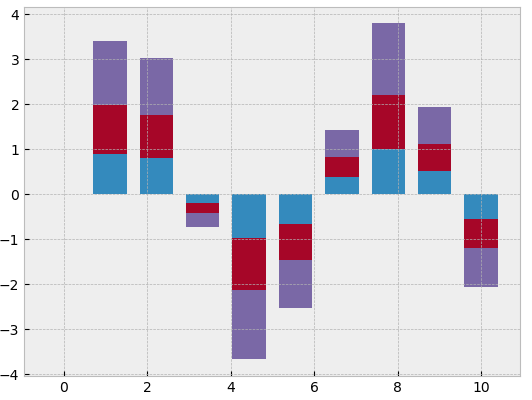通过调整条形图的宽度和横坐标来实现绘制并列柱状图

plt.style.use('bmh')
x=np.linspace(0,10,10)
plt.bar(x,np.sin(x),width=0.3)
plt.bar(x+0.3,1.2*np.sin(x),width=0.3)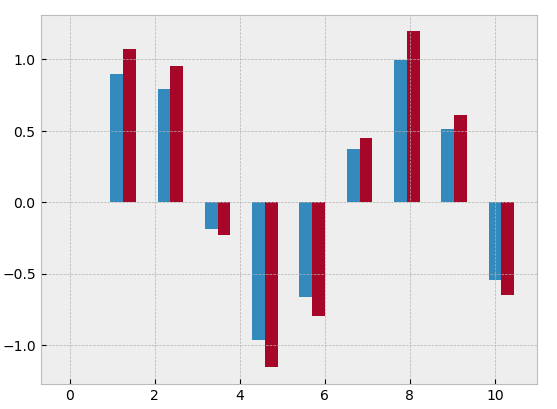本文在此只对几种简单的绘制方法做介绍，了解更多方法可查询官网或参考其他博文。

### 5.2 饼图

绘制饼图，我们使用plt.pie()函数，其常用参数表如下

plt.pie(x,explode,labels,colors,autopct,pctdistance=0.6,labeldistance=1.1,radius)

参数名称说明参数名称说明
x表示用于绘制饼图的数据explode表示指定项离饼图圆心为n个半径
labels指定每一项的名称colors表示饼图颜色
autopct指定数值精度，数据会自动换算成百分比pctdistance指定每一项的比例和距离饼图圆心n个半径
x=np.linspace(1,10,5)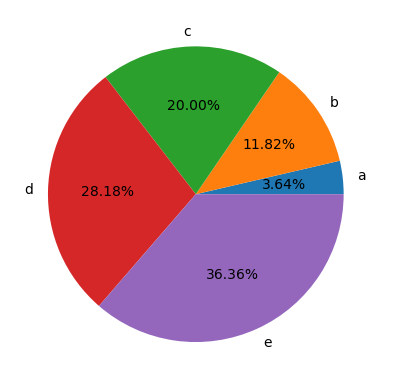如果要创建环形饼图，在创建饼图后再创建一个纯白色的半径更小的饼图即可。

x=np.linspace(1,10,5)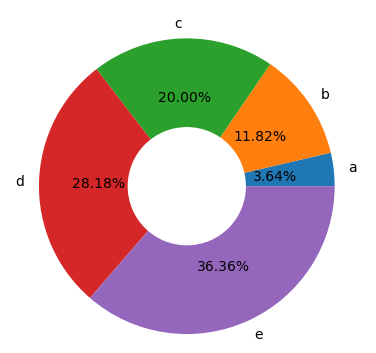了解更多创建饼图的方法可查询官网和参考其他博文。

## 6.密度图与等高线图

有时在二维图上用等高线图或色彩图来表示三维数据是不错的方法。Matplotlib提供了三个函数来解决这个问题：用plt.contour()画等高线图、用plt.contourf()画带有填充色的等高线图（filled contour plot）的色彩、用plt.imshow()显示图形。

### 6.1 三维函数的可视化

用函数 z = f ( x , y ) z=f(x,y) 演示等高线图，按照下面的方式生成函数：

def f(x,y):
return np.sin(x)**10+np.cos(10+y*x)*np.cos(x)


等高线图可以用plt.contour()函数来创建。它需要三个参数：x轴，y轴和z轴三个坐标轴的网格数据。x轴与y轴表示图形中的位置，而z轴将通过等高线的等级来表示。使用np.meshgrid()函数来准备这些数据是一个比较简单的方法，它可以从一维数组构建二维网格数据：

x=np.linspace(0,5,50)
y=np.linspace(0,5,40)
X,Y=np.meshgrid(x,y)
Z=f(X,Y)


下面我们来创建等高线图：

plt.contour(X,Y,Z,6)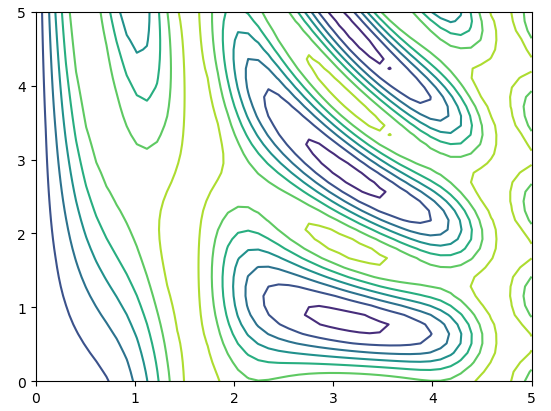其中，6表示将数据范围等分为6份。此外，我们可以通过使用colors参数与cmap参数来设置等高线图的颜色，特别需要注意的是，在只使用一种颜色时，默认使用虚线表示负数，使用实线表示正数。

接下来，我们使用plt.contourf()来创建填充的等高线图，它的语法与plt.contour()是一样的。另外我们可以使用plt.colorbar()来创建一个表示图形各种颜色对应标签信息的颜色条。

plt.contourf(X,Y,Z,6)
plt.colorbar()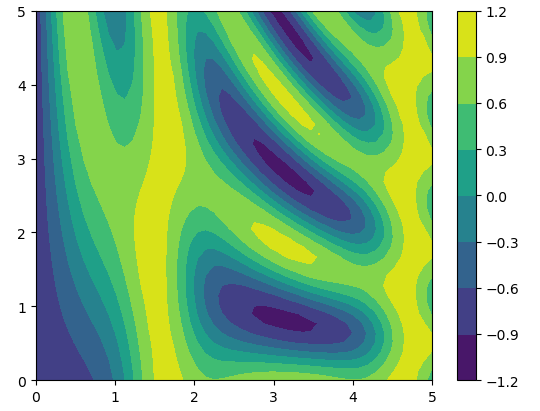通过颜色条，我们可以清晰地看出，黄色的部分对应的数值最高，是“波峰(peak)”；深蓝色的部分对应的数值最低，是“波谷(valley)”。

我们还可以通过plt.imshow()函数来将二维数组渲染成渐变图。

plt.imshow(Z,extent=[0,5,0,5],origin='lower')
plt.colorbar()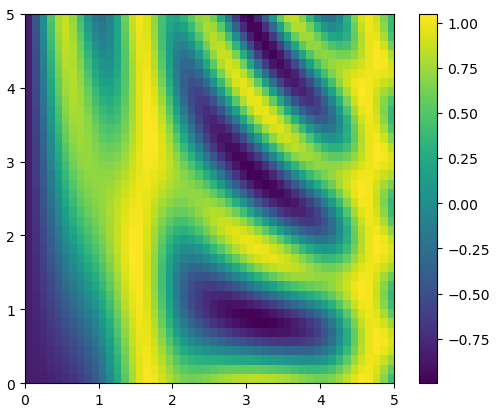使用plt.imshow()需要注意以下几点：1.它不支持用x轴和y轴数据设置网格，而是必须通过extent参数设置图形的坐标范围[xmin,xmax,ymin,ymax]。2.默认使用标准的图形数组定义，即原点位于左上角，这一点在显示网格数据图形时必须调整。3.plt.imshow()会自动调整坐标轴的精度以适应数据显示。可以通过plt.axis(aspect='image')来设置x轴与y轴的单位。

另外，再介绍一种方法，将等高线图与彩色图组合起来。

contours=plt.contour(X,Y,Z,3,colors='black')
plt.clabel(contours,inline=True,fontsize=8) #在等高线图中加入label
plt.imshow(Z,extent=[0,5,0,5],origin='lower',alpha=0.5)
plt.colorbar()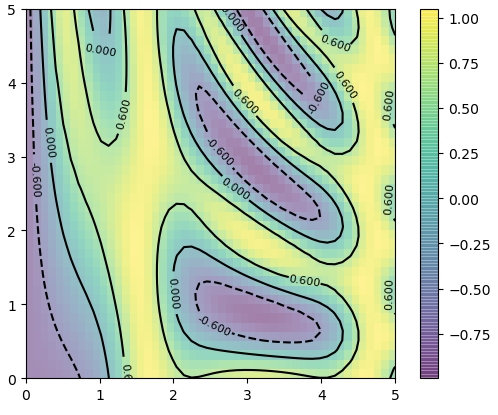基于这几种方式，使用二维图表示三维数据的方法就有很多种可能了，想要了解更多的读者可以查询官网或是参考其他博文。

## 7.频次直方图、数据区间划分与分布密度

### 7.1 频次直方图

直接引入实例来讲解

x1=np.random.normal(0,0.8,1000)
x2=np.random.normal(-2,1,1000)
x3=np.random.normal(3,2,1000)
kwargs=dict(histtype='stepfilled',alpha=0.3,density=True,bins=40,edgecolor='none')
plt.hist(x1,**kwargs)
plt.hist(x2,**kwargs)
plt.hist(x3,**kwargs)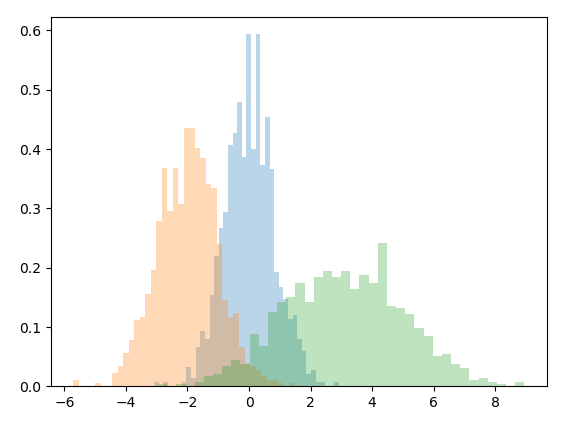如上例所示，使用这样的直方图可以很好地帮助我们分析数据的分布情况，并对不同数据集的分布特征进行对比。现简要介绍用到的几个参数。

histtype是使用直方图的类型，常用类型有：‘bar’，‘barstacked’，‘step’，‘stepfilled’。

alpha指的是绘制直方图的透明度。

density是bool类型，若其值为True，则将频数转化为频率绘制直方图，即将频数归一化。

bins是指直方图柱形的数量，即区间内的分组个数。

edgecolor即是边缘颜色。

常用的其他参数还有’color’（直方图颜色），‘bottom’（y轴的起始位置），‘orientation’（直方图显示方式horizontal or vertical）等，具体方法可查询官网或参考其他博文。例如：【Matplotlib】（五）直方图(频数统计图).

另外，提供仅计算出每段区间样本数的代码：

counts,bin_edges=np.histogram(x1,bins=20)
print(counts)


### 7.2 二维频次直方图与数据区间划分

就像将一维数组分为区间创建一维频次直方图一样，我们也可以将二维数组按照二维区间进行切分，来创建二维频次直方图。下面来简单介绍几种创建二维频次直方图的方法。

#### 7.2.1 二维频次直方图plt.hist2d()

mean=[0,0]
cov=[[1,1],[1,2]]
x,y=np.random.multivariate_normal(mean,cov,10000).T

plt.hist2d(x,y,bins=30)
plt.colorbar()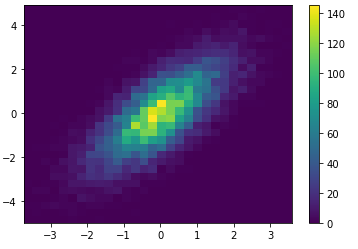另外，二维频次直方图也可以提供仅计算出每段区间样本数的代码：

counts,xedges,yedges=np.histogram2d(x,y,bins=20)
print(counts)


#### 7.2.2 六边形区间划分plt.hexbin()

二维频次直方图是正方形分割的，还有一种常用的方式是用正六边形分割。

plt.hexbin(x,y,gridsize=30)
plt.colorbar()
plt.xlim(-3.5,3.5)
plt.ylim(-4.5,4.5)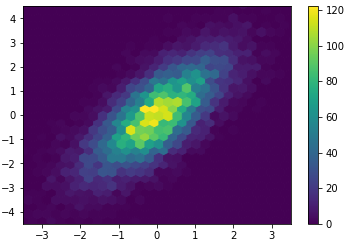#### 7.2.3 核密度估计（KDE方法）

KDE方法是一种评估多维数据分布密度的常用方法，在scipy.stats中有一个简单快速实现KDE的方法，现在来举例演示：

from scipy.stats import gaussian_kde

#拟合数组维度[Ndim,Nsamples]
data=np.vstack([x,y])
kde=gaussian_kde(data)

#用一对规则的网格数据进行拟合
xgrid=np.linspace(-3.5,3.5,40)
ygrid=np.linspace(-6,6,40)
Xgrid,Ygrid=np.meshgrid(xgrid,ygrid)
Z=kde.evaluate(np.vstack([Xgrid.ravel(),Ygrid.ravel()]))

#画出结果图
plt.imshow(Z.reshape(Xgrid.shape),origin='lower',aspect='auto',extent=[-3.5,3.5,-6,6])
plt.colorbar()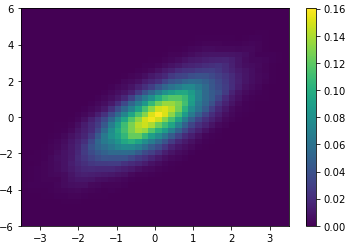用Matplotlib做KDE的可视化图的过程比较繁琐，之后会介绍的Seaborn库中会提供更简洁的方法。

## 8.可视化异常处理

误差在测量过程中是不可避免的，如果仅有对测量数据的可视化，那么就无法直观的展示数据的可信程度或说难以直观的感受到与理论值的差异。在数据可视化的结果中用图形展示误差，就可以提供更充分的信息。

### 8.1 基本误差线

x=np.linspace(0,10,50)
dy=0.8
y=np.sin(x)+dy*np.random.randn(50)

plt.errorbar(x,y,yerr=dy,fmt='o',color='k',ecolor='lightblue',elinewidth=3,capsize=0)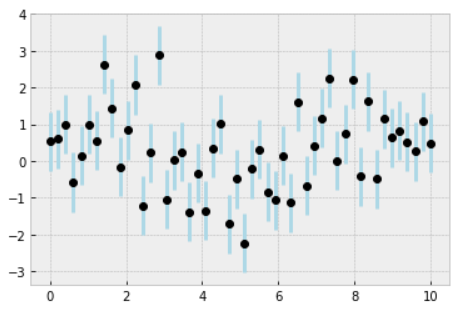在此仅介绍简单的绘制方法，更多选项和参数的信息可参考plt.errorbar()的程序文档。

### 8.2 连续误差

有时我们需要显示连续变量的误差。Matplotlib中并没有内置的简便方法可以解决这个问题，但是通过plt.fill_between()来解决也不是难题。
在这里，我们仅展示一个例子：

注：以下代码参考博文【Python实例第36讲】一个高斯过程回归的例子.

from sklearn.gaussian_process import GaussianProcessRegressor
from sklearn.gaussian_process.kernels import RBF, ConstantKernel as C
def f(x):
return x * np.sin(x)
kernel = C(1.0, (1e-3, 1e3)) * RBF(10, (1e-2, 1e2))
gp=GaussianProcessRegressor(kernel=kernel, n_restarts_optimizer=9)
X = np.atleast_2d([1., 3., 5., 6., 8.]).T
y = f(X).ravel()
x = np.atleast_2d(np.linspace(0, 10, 1000)).T
gp.fit(X, y)
y_pred, sigma = gp.predict(x, return_std=True)
plt.figure()
plt.plot(X, y, 'r.', markersize=10, label=u'Observations')
plt.plot(x, y_pred, 'b-', label=u'Prediction')
plt.fill(np.concatenate([x, x[::-1]]),
np.concatenate([y_pred - 1.9600 * sigma,
(y_pred + 1.9600 * sigma)[::-1]]),
fc='lightblue', ec='None', label='95% confidence interval')
plt.xlabel('$x$')
plt.ylabel('$f(x)$')
plt.ylim(-10, 20)
plt.legend(loc='upper left')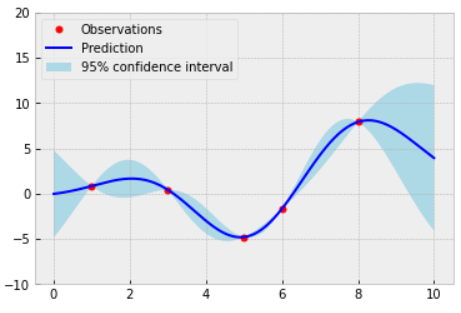我们使用了高斯过程回归方法，从图中可以看出，在接近观测点的位置，模型受到强约束，拟合误差很小，非常接近真实值；而在远离样本的区域，模型不受约束，误差不断增大。

后续我们会介绍Seaborn库中实现连续误差线的简便方法。

## 后记

以上便是《使用Matplotlib进行数据可视化（一）》的全部内容了，这算是笔者自己的学习记录，也算是个人经验的一些分享，我会不定期更新这一系列的内容，希望能够给各位读者带来一定的帮助。

展开全文python 编程语言
• Python 入门 matplotlib数据可视化基础【二】分析特征之间的关系【散点&折线图】绘制散点图适用场景scatter函数绘制折线图plot函数综合实现 分析特征之间的关系【散点&折线图】 绘制散点图 适用场景 散点图是以一个...
• 导读：Matplotlib是建立在NumPy数组上的一个多平台数据可视化库。在2002年，约翰·亨特（John Hunter）提出Matplotlib，最初的构思是设计为IPython的...python 人工智能 编程语言
• 数据科学---使用Matplotlib进行数据可视化python
• %matplotlib inline 这条命令的作用是将 Matplotlib 绘制的图形嵌入在当前页面中。 在桌面环境中绘图时，不需要添加此命令，而是在全部绘图代码之后追加plt.show() 使用 Matplotlib 提供的面向对象 API，需要导入...python
• 进行数据可视化（pyecharts，matplotlib……） 我们进入该网址使用快捷键F12浏览源代码 经过漫长的寻找我们找到了所需要的数据： 经过整理，我们发现它的数据是这样的： [{{“provinceName”:“黑龙江省”,...
• Python数数据据可可视视化化教教程程之之Matplotlib实实现现各各种种图图表表实实例例 这篇文章主要给大家介绍了关于Python数据可视化教程之利用Matplotlib实现各种图表的相关资料文中通过示例 码介绍的非常详细对...
• 在完成数据收集和统计工作之后，利用Matplotlib库将数据进行可视化展示，最后得到的结果不但美观，还可以让人们非常直观快速的从数据中获取信息.。python
• 使用Matplotlib库以线图可视化使用Matplotlib库使用散点图进行可视化使用Matplotlib库以直方图可视化使用Matplotlib库使用条形图进行可视化使用Matplotlib库对子图进行可视化资源利用该示例的屏幕截图： 数据集中的...Python
• 点击上方“小白学视觉”，选择加"星标"或“置顶”重磅干货，第一时间送达作者：橡鱼转自：Datawhale数据可视化，就是指将结构或非结构数据转换成适当的可视化图表，然后将隐藏在数据中的信息直接展现于人们面前。...
• 在学习Python时到数据可视化时，遇到了一些问题，代码输入后运行报错，无法画出图像。在此当做学习笔记记录一下解决方法，供大家参考。 代码如下： import numpy as np import matplotlib.pyplot as plt import ...vscode ide
• Matplotlib数据可视化 1. 通过Matplotlib绘制各种图形 1.1 绘制折线图 from matplotlib import pyplot as plt # import matplotlib.pyplot as plt import matplotlib import numpy as np # 通过Numpy设置x轴和y轴 x ...python
• Matplotlib基础03：利用已学知识绘制boston房价的影响因素表 波士顿房价数据集介绍 散点图描绘这些因素对房价的影响 1.我们先下载数据集 boston = tf.keras.datasets.boston_housing #加载数据，分训练集与测试集...
• importmatplotlib.pyplotaspltplt.rcParams['font.sans-serif']=['simhei']#用于正常显示中文标签plt.rcParams['axes.unicode_minus']=False#用于正常显示负号plt.plot()绘制线性二维图，折线图注意：如果向plot()...
• 思来想去，虽然很忙，但还是挤时间针对这次肺炎疫情写个Python大数据分析系列博客，包括网络爬虫、可视化分析、GIS地图显示、情感分析、舆情分析、主题挖掘、威胁情报溯源、知识图谱、预测预警及AI和NLP应用等。...
• 大家好，我是 阳哥。Matplotlib 是 Python 数据可视化中不可或缺的一个可视化库，「Python数据之道」之前也给大家分享了一系列 Matplotlib 从基础到进阶的内容，...编程语言 数据挖掘 python
• 数据可视化在机器学习和数据科学中是很重要的组成部分。在数据分析阶段，数据可视化能够帮助我们理解洞察数据间关系；在算法调试阶段，数据可视化能够发现问题，优化算法；在项目总结阶段，数据可视化能够展示项目...python
• 第五章 使用NumPy和Matplotlib进行数据可视化 目录 一、Matplotlib 二、使用NumPy和Matplotlib实现可视化 三、单线图（Single Line Plots） 四、多线图（Multiline Plots） 五、网格、轴和标签（Grid，Axes，and ...人工智能 python java 编程语言
• matplotlib对于数据可视化非常重要，它完全封装了MatLab的所有API，在python的环境下和Python的语法一起使用更是相得益彰。 一、库的安装和环境的配置windows下：py -3 -m pip install matplotliblinux下：pytmatlab python
• 利用ipython --pylab，可以进入PyLab模式，已经导入了matplotlib库与相关软件包（例如Numpy和Scipy)，额可以直接使用相关库的功能。 本文作为学习过程中对matplotlib一些常用知识点的整理，方便查找。 这样IPython...
• 本篇利用python中的matplotlib库进行简单的数据可视化分析，数据来源于博客园中《2020年4月中国编程语言排行榜程序员工资统计，人工智能工资大跌》 数据描述 数据处理过程 饼状图：引入matplotlib库之后，设置中文...python
• import matplotlib.pyplot as plt edu = ['0.2515','0.3724','0.3336','0.0368','0.0057'] labels = ['中专','大专','本科','硕士','其他'] plt.rcParams['font.sans-serif'] = ['Arial Unicode MS']#使图像中可以...
• 他们用数据可视化的方式绘画，试图展现数据内隐藏的模式或表达对数据的见解。更有趣的是，一旦接触到任何可视化的内容、数据时，人类会有更强烈的知觉、认知和交流。 数据可视化是数据科学家工作中的重要组成部分。...
• 2、外观和个性—> 3、字体—> 4、复制.tff文件（复制你想添加进matplotlib里的） 自己网上下载 字由 第②步 设置matplotlib 1、找到matplotlib包的位置,添加tff 右键你的python快捷方式，打开文件所在文件夹，如果......